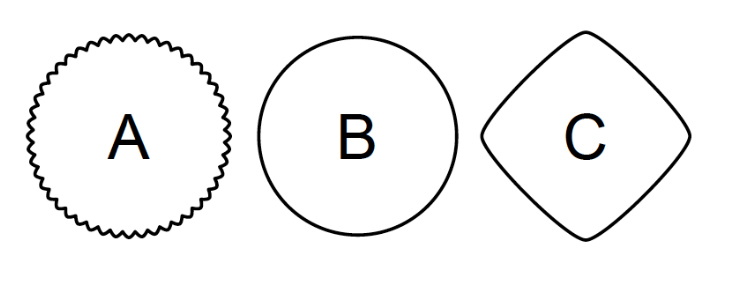Natural Sciences

Associate Professor Klas Modin

Chalmers University of Technology

## Will develop the mathematics of shapes

In school, we learn how to calculate the distance between two points using coordinates and  the Pythagorean theorem. Wallenberg Academy Fellow Klas Modin has developed mathematics that can calculate distances in a more abstract world and can be used to analyze complicated shapes, such as that of a protein or organ.

In mathematics, a small distance is synonymous with a small difference. Using the mathematics called shape analysis, researchers can study whether the difference between figures A and B below is smaller than the difference between B and C.The mathematically correct answer depends on which concept of distance is used in the analysis and how sensitive it is to interference.

Associate Professor Klas Modin from Chalmers University of Technology is developing new mathematical techniques to study distance in shape analysis, building upon mathematical methods in which a transformation group deforms an object. The distance between shapes A and B is then defined as the shortest transformation route that takes A to B.

In shape analysis, a shape can, for example, be a surface, an image, electrical field or a particle cloud in physics.  The mathematical techniques being developed by Klas Modin will be valuable tools for researchers who study the shape of proteins through cryo-electron microscopy, or for physicians who use MRIs to search for shape deviations in organs, which could be tumors.

Foto: Marcus Marcetic